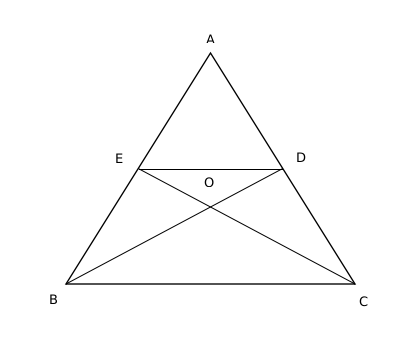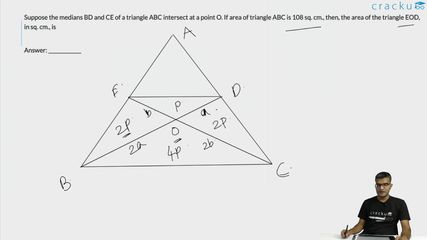Question 5

# Suppose the medians BD and CE of a triangle ABC intersect at a point O. If area of triangle ABC is 108 sq. cm., then, the area of the triangle EOD, in sq. cm., is

SolutionArea of ABD : Area of BDC = 1:1

Therefore, area of ABD = 54

Area of ADE : Area of EDB = 1:1

Therefore, area of ADE = 27

O is the centroid and it divides the medians in the ratio of 2:1

Area of BEO : Area of EOD = 2:1

Area of EOD = 9

### View Video Solution##### Suriya Narayanan

2 months, 1 week ago

Why the answer is not 12 as the triangles can be equally divided using the centroid.
Now each triangle has 36cm2. And the triangle are in the ratio of 2:1, which makes the required area EOD as 12cm2

##### susheela rath

7 months, 1 week ago

Ytherwd2

##### Deep Sheth

11 months, 1 week ago

What a solution. Excellent teaching style Maruti sir. Hats off

##### susheela rath

7 months, 1 week ago

Heheh3g

##### susheela rath

7 months, 1 week ago

Kutudu harami